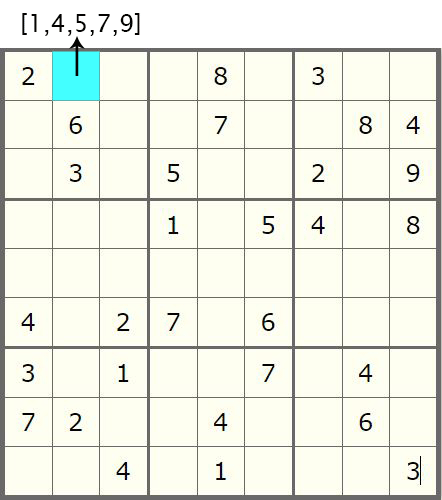﻿ 使用Python进行数独求解详解(二)_python_脚本之家
python# 使用Python进行数独求解详解(二)

## 2. 前文回顾## 3. 减少非比要的迭代次数

```# Solve Sudoku using backtracking
def solve(board):
blank = findEmpty(board)
if not blank:
return True
else:
row, col = blank

for i in range(1,10):
if isValid(board, i, blank):
board[row][col] = i

if solve(board):
return True

board[row][col] = 0
return False
```### 3.1 生成候选值字典

```# Store in a dictionary the legitimate
# values for each individual cell
def cacheValidValues(board):
cache = dict()
for i in range(9):
for j in range(9):
if board[i][j] == 0:
cache[(i,j)] = allowedValues(board,i,j)
return cache
```

### 3.2 生成候选值列表

```def allowedValues(board,row,col):

numbersList = list()

for number in range(1,10):

found = False
# Check if all row elements include this number
for j in range(9):
if board[row][j] == number:
found = True
break
# Check if all column elements include this number
if found == True:
continue
else:
for i in range(9):
if board[i][col] == number:
found = True
break

# Check if the number is already included in the block
if found == True:
continue
else:
rowBlockStart = 3* (row // 3)
colBlockStart = 3* (col // 3)

rowBlockEnd = rowBlockStart + 3
colBlockEnd = colBlockStart + 3
for i in range(rowBlockStart, rowBlockEnd):
for j in range(colBlockStart, colBlockEnd):
if board[i][j] == number:
found = True
break
if found == False:
numbersList.append(number)
return numbersList
```

### 3.3 函数调用

```def solveWithCache(board, cache):
blank = findEmpty(board)
if not blank:
return True
else:
row, col = blank

for value in cache[(row,col)]:
if isValid(board, value, blank):
board[row][col] = value

if solveWithCache(board, cache):
return True

board[row][col] = 0
return False
```

```The execution time of above program is : 15.41820478439331 s
```